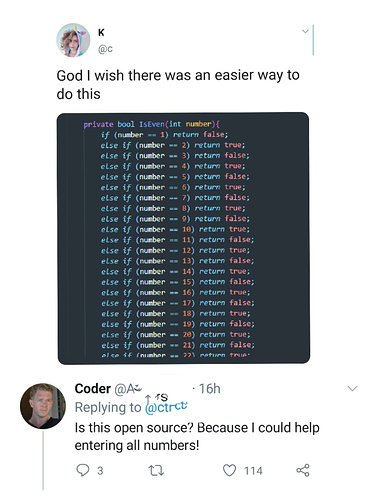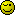# How to check if a number is odd?

Based on this:The idea here that this is obviously wrong because there are better ways to do this. So, what ways are there?Disclaimer: This topic is just meant as discussion, I know several answers, just wondering what others come up with.

3 Likes

Easiest way would be to use the modulus operator:

``````const isEven = num => num%2 === 0;

isEven(199)
// false

isEven(200)
// true
``````
3 Likes

What he said. Time tested method. Divide by two and if there’s a remainder, it’s odd.

yep, I agree modulo - in php…
fmod(x,y) returns 0 if no remainder
so divide by 2
so fmod(10, 2) returns 0
whereas
fmod(7, 2) returns 1

and since it’s just a discussion I guess any string resulting from a division by 2, if the result was even would only contain 0s after the ‘.’ or no ‘.’ at all

however I am not sure what happens with 0 as one of the arguments - is 0 an odd number or even ?

Just for fun, apparently if it is a whole number (not a decimal) if the last digit is 0,2,4,6 or 8 then it is even, any other digit and it’s odd

so even without a calculator …
12345678901234567890 is even, but
12345678901234567891 is odd
just check the last digit !

You might want to define “even” and “odd” as, at least on the internet, there seems to be some debate.

how can there be debate about this? they are clearly defined mathematical conceptsLet’s go with

even = integer that can be divided exactly by 2
odd = integer that can not be divided exactly by 2

4 Likes

``````
function isEven (\$n)
{
if (gettype (\$n) != "integer") return false;
return ((\$n & 1) == 0) ? 1 : 0;
}
``````

Edit: I don’t know why my brain turned the question into checking if a number is even rather than odd, but the switch is… apparent.Using the venerable isOdd package:

``````const isOdd = require('is-odd');

console.log(isOdd('1')); //=> true
console.log(isOdd('3')); //=> true

console.log(isOdd(0)); //=> false
console.log(isOdd(2)); //=> false
``````

Before you knock it, bear in mind that it has 483k weekly downloads…

Here is how the experts do it: https://github.com/jonschlinkert/is-odd/blob/master/index.js

2 Likes

In PHP.

``````if(\$n & 1){}
``````

If it’s `true`, it’s odd.

2 Likes

In python you can write it as
Odd=numbers which are not divisible by 2

Program Solution

``````x=input("enter any number")
if(x/2!=0);
print("number is odd")
else
print("number is even")
``````
1 Like

Really inefficient, but works too:

``````function isOdd(\$n): bool {
while (\$i >= 0) {
\$i -= 2;
}
return \$i === -1;
}
``````

Fun with Php

``````<?php

\$arr = range(3,9000,2);
\$num = 9;

if (in_array(\$num, \$arr)){
echo "Num is odd";
}
``````
1 Like

Oh, oh, generators!

``````<?php
function isOdd(\$i) {
if (\$i === 0) { return false; }

\$sign = \$i > 0 ? 1 : -1;

\$oddNumberGenerator = function() use (\$sign) {
\$i = \$sign; // start at -1 when \$i is positive, at 1 when \$i is negative
while (true) {
\$i += \$sign * 2; // add 2 when \$i is positive, substract 2 when \$i is negative
yield \$i;
}
};

foreach (\$oddNumberGenerator() as \$oddNumber) {
if (\$i === \$oddNumber) { return true; }
if (\$i === \$oddNumber + 1) { return false; }
}
}
``````

It’s horribly ineffecient. Just deciding whether `99999999` is odd takes 10 seconds on https://repl.it/languages/php2 Likes

Wait, wait, how bout this?

``````<?php

\$num =216259;
\$x = substr(\$num, -1);

if  (\$x == 1 || \$x == 3 ||\$x == 5 || \$x == 9){
echo 'Num is odd';
}
``````
1 Like

Or this``````function isOdd(\$i) {
return substr((string) (\$i / 2), -2) === '.5';
}
``````
2 Likes

You didn’t specify which programming language. In many languages, the likely most efficient method would be to use a bitwse operator. For example, in Javascript, like so:

``````const isOdd = value & 1
``````

Here we’re ANDing the value with 1, which will return 1 (truthy) if and only if the right-hand bit of value is also 1. Odd values have that right-hand bit set while even values (and zero) don’t.

And then of course using recursion:

``````function isOdd (value) {
return value !== 0 && (value === -1 || isOdd(Math.abs(value) - 2))
}
``````
2 Likes

This topic was automatically closed 91 days after the last reply. New replies are no longer allowed.